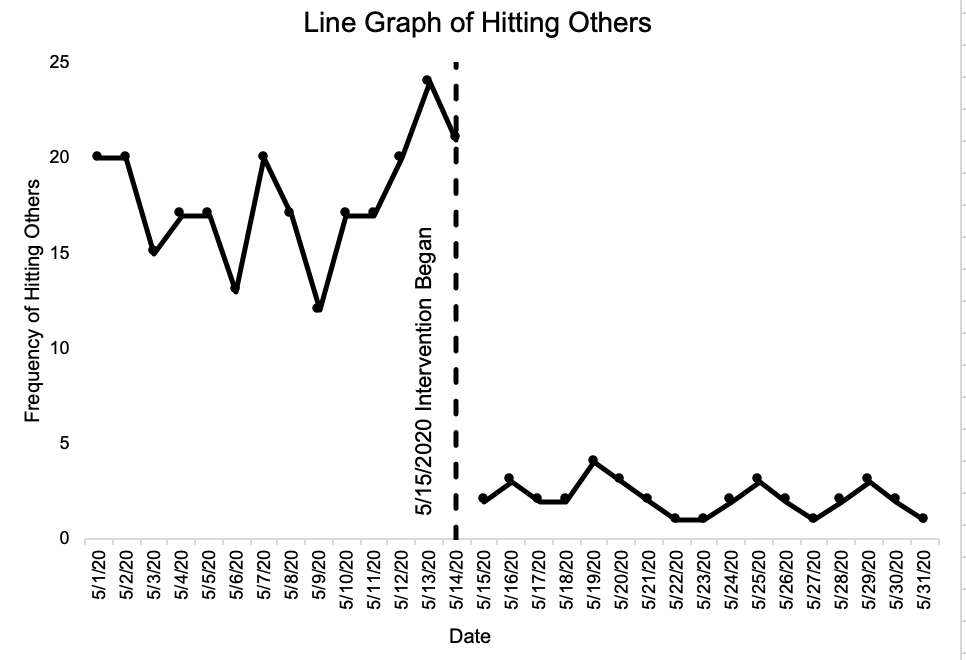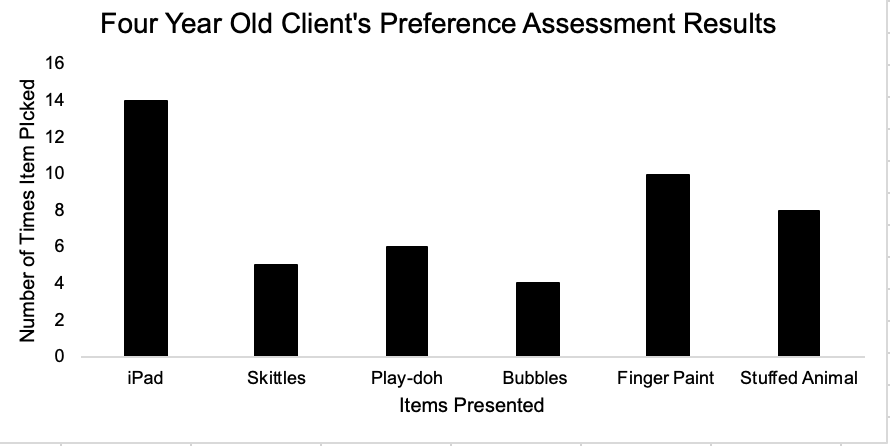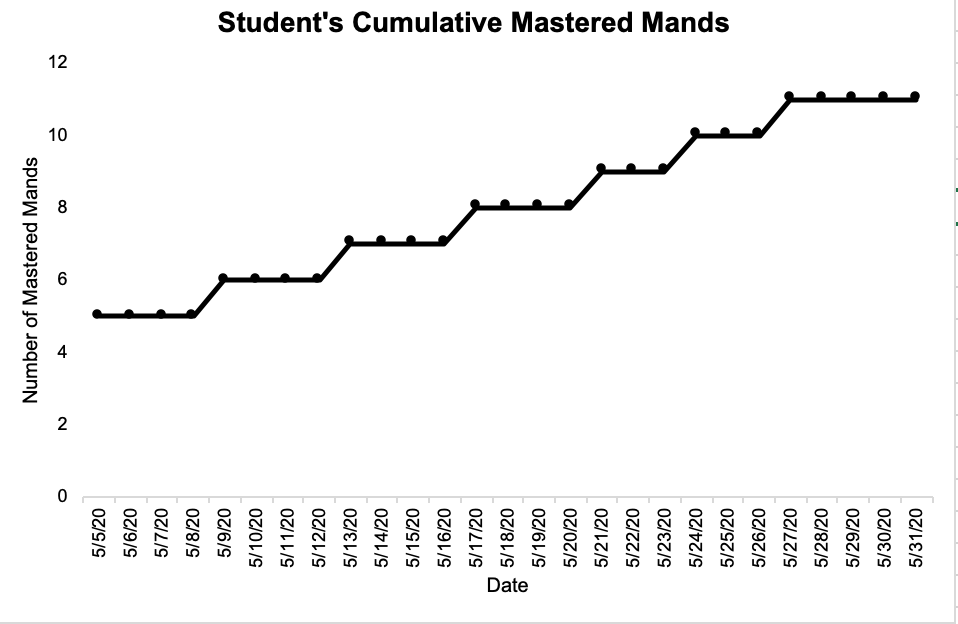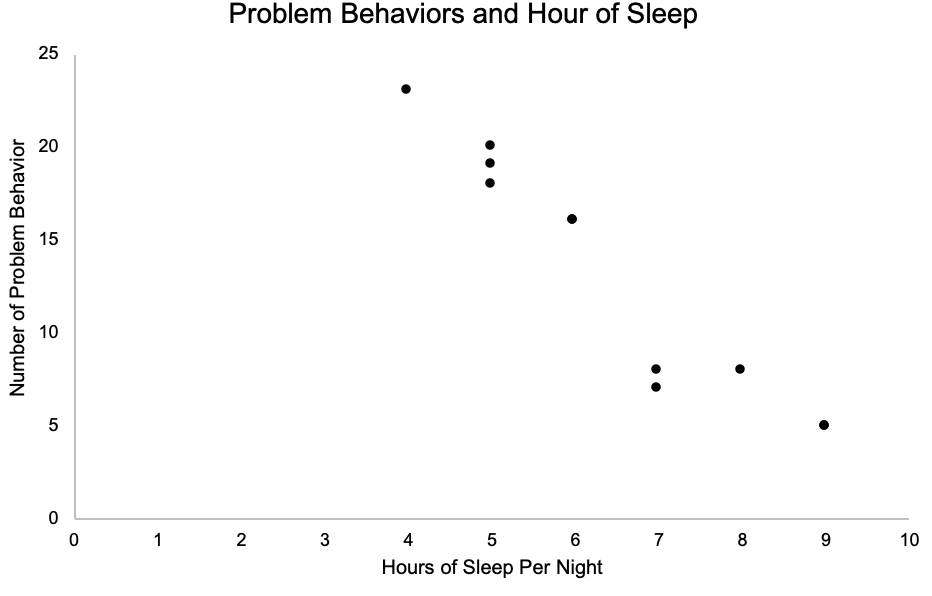## C-10: Graph data to communicate relevant quantitative relations (e.g., equal-interval graphs, bar graphs, cumulative records) ©

Target Terms: Equal-interval graph, Bar graph, Cumulative records, Semilogarithmic Chart (Standard Celeration Chart), ScatterplotEqual-Interval Graph

Definition: Graphs where the distance between two consecutive points on both the X and Y axis represent the same value (e.g., the X axis and Y axis are both intervals of five).

Example of Equal-Interval Graphs: Line graphs, Bar graphs, Cumulative records, and Scatterplots.

Example of Non-Equal-Interval Graphs: Semilogarithmic Charts (Standard Celeration Chart).

Line Graph

Definition: A graph based on the Cartesian plane where a two-dimensional area is formed by the intersection of two or more lines forming a data path.

Example in a clinical context: A behavior analyst creates a line graph that displays baseline data and intervention data on the target behavior of hitting others.

Why it matters: Line graphs should be used when you want your data to communicate quantitative relations such as time or the order of responses in a sequence. Line graphs can include multiple data paths for different behaviors.Bar Graph

Definition: A graph based on the Cartesian plane where there are no distinct data points representing responses through time.

Example in a clinical context: A behavior analyst displays data from a preference assessment that was conducted with a child.

Why it matters: Bar graphs are used to display a set of data that are not related to each other.Cumulative Records

Definition: A graph that displays the cumulative number of responses emitted are represented on the vertical axis, where the steeper the slope of the response the greater the response rate.

Example in a clinical context: A teacher is conducting a manding program with a student and uses a cumulative record to display the student’s mastered mands.

Why it matters: Cumulative records demonstrate response frequency over a period of time, which helps to quickly conceptualize the rate at which a learner is gaining new skills.Semilogarithmic Charts (Standard Celeration Chart)

[Example SCC coming soon!]

Scatterplot

Definition: A graph that shows the relative distribution of individual measures in a data set (e.g., aggression, sleep, etc.).

Example in clinical context: A behavior analyst creates a scatterplot of a client’s aggressive behavior to determine any temporal patterns between aggression and time of day.

Why it matters: Scatterplots communicates temporal relations of a behavior of interest if one exists.